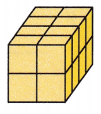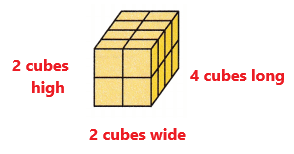# McGraw Hill Math Grade 5 Chapter 10 Lesson 8 Answer Key Counting Unit Cubes and Volume

All the solutions provided in McGraw Hill Math Grade 5 Answer Key PDF Chapter 10 Lesson 8 Counting Unit Cubes and Volume are as per the latest syllabus guidelines.

## McGraw-Hill Math Grade 5 Answer Key Chapter 10 Lesson 8 Counting Unit Cubes and Volume

Count

Count the cubes in each object. Write the total.

Question 1.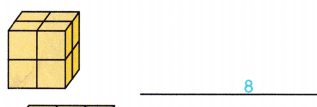Question 2.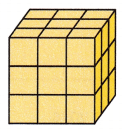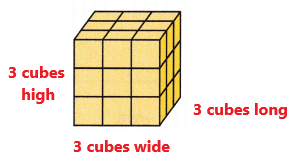Explanation:
In the above object we can observe 3 rows. There are total 3 cubes in each row.
3 + 3 + 3 = 9 cubes
In one layer there are 9 cubes.
Now we have to add the number of cubes in each layer.
There are three layers in the above object.
9 + 9 + 9 = 27 cubes
The total number of cubes in the above object are 27.

Question 3.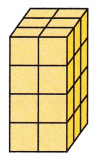Explanation:
In the above object we can observe 3 rows. There are total 2 cubes in each row.
2 + 2 + 2 = 6 cubes
In one layer there are 6 cubes.
Now we have to add the number of cubes in each layer.
There are four layers in the above object.
6 + 6 + 6 + 6 = 24 cubes
The total number of cubes in the above object are 24.

Question 4.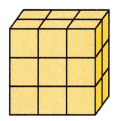Explanation:
In the above object we can observe 2 rows. There are total 3 cubes in each row.
3 + 3 = 6 cubes
In one layer there are 6 cubes.
Now we have to add the number of cubes in each layer.
There are three layers in the above object.
6 + 6 + 6 = 18 cubes
The total number of cubes in the above object are 18.

Question 5.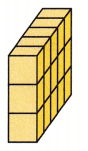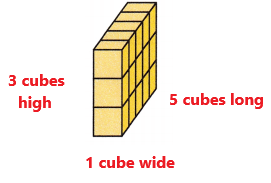Explanation:
In the above object we can observe 5 rows. There are total 1 cubes in each row.
1 + 1 + 1 + 1 + 1 = 5 cubes
In one layer there are 5 cubes.
Now we have to add the number of cubes in each layer.
There are three layers in the above object.
5 + 5 + 5 = 15 cubes
The total number of cubes in the above object are 15.

Question 6.